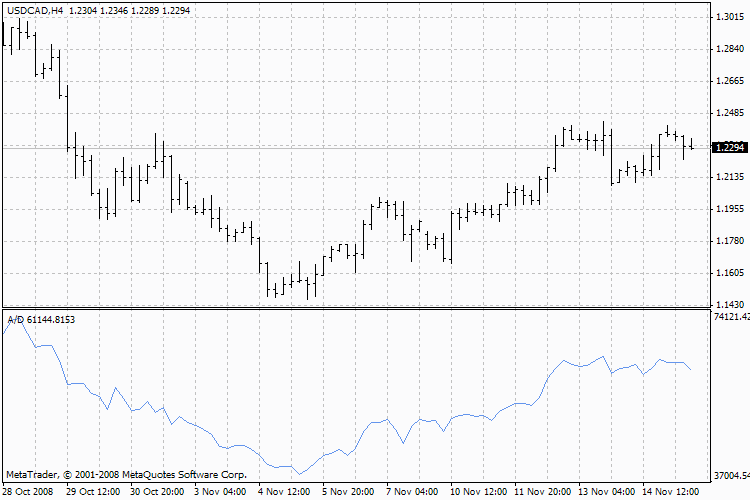Watch how to download trading robots for free

Interesting script?
So post a link to it -
let others appraise it

You liked the script? Try it in the MetaTrader 5 terminal# Accumulation Distribution - indicator for MetaTrader 4

Views:
19647
Rating:
votes: 11
Published:
2005.11.29 11:51
Updated:
2016.11.22 07:32

Accumulation/Distribution Technical Indicator is determined by the changes in price and volume. The volume acts as a weighting coefficient at the change of price — the higher the coefficient (the volume) is, the greater the contribution of the price change (for this period of time) will be in the value of the indicator.Accumulation/Distribution, A/D

In fact, this indicator is a variant of the more commonly used indicator On Balance Volume. They are both used to confirm price changes by means of measuring the respective volume of sales.

When the Accumulation/Distribution indicator grows, it means accumulation (buying) of a particular security, as the overwhelming share of the sales volume is related to an upward trend of prices. When the indicator drops, it means distribution (selling) of the security, as most of sales take place during the downward price movement.

Divergences between the Accumulation/Distribution indicator and the price of the security indicate the upcoming change of prices. As a rule, in case of such divergences, the price tendency moves in the direction in which the indicator moves. Thus, if the indicator is growing, and the price of the security is dropping, a turnaround of price should be expected.

Calculation

A certain share of the daily volume is added to or subtracted from the current accumulated value of the indicator. The nearer the closing price to the maximum price of the day is, the higher the added share will be. The nearer the closing price to the minimum price of the day is, the greater the subtracted share will be. If the closing price is exactly in between the maximum and minimum of the day, the indicator value remains unchanged.

A/D(i) =((CLOSE(i) - LOW(i)) - (HIGH(i) - CLOSE(i)) * VOLUME(i) / (HIGH(i) - LOW(i)) + A/D(i-1)

Where:
A/D(i) — importance of the Indicator of the Accumulation/Distribution for the current bar;
CLOSE(i) — the price of the closing the bar;
LOW(i) — the minimum price of the bar;
HIGH(i) — the maximum price of the bar;
VOLUME(i) — volume;
A/D(i-1) — importance of the Indicator of the Accumulation/Distribution for previous bar.

Technical Indicator Description

Full description of A/D is available in the Technical analysis: Accumulation/Distribution

Translated from Russian by MetaQuotes Software Corp.
Original code: https://www.mql5.com/ru/code/7800Accelerator Oscillator (AC)

Acceleration/Deceleration Indicator (AC) measures acceleration and deceleration of the current driving force.MA2CCI

Sample Trading Sistem for cross 2MA & CCIAlligator

Alligator indicator is a combination of Balance Lines that use fractal geometry and nonlinear dynamics.Average True Range, ATR

Average True Range Indicator (ATR) is an indicator that shows volatility of the market.